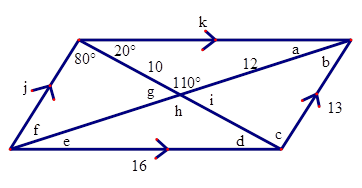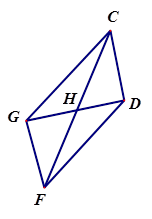# Parallelograms Warm Up

Author:
powere

## Use the diagram below to fill in for the missing lengths and angles. Please ignore the units when entering an answer.1. A = _____ ﻿B = _____ ﻿C = _____ ﻿D = _____ ﻿ E = _____ ﻿ F = _____ ﻿G = _____ ﻿H = _____ ﻿ I = _____ ﻿ J = _____ ﻿K = _____2. CH = ____

Select all that apply
• A
• B
• C
• D3. GF is parallel to _____

Select all that apply
• A
• B
• C
• D4. <DCG is congruent to _____

Select all that apply
• A
• B
• C
• D5. DC = _____

Select all that apply
• A
• B
• C
• D6. <DCG is supplementary to ____ and ____

Select all that apply
• A
• B
• C
• D7. Triangle HGC is congruent to _____

Select all that apply
• A
• B
• C
• D